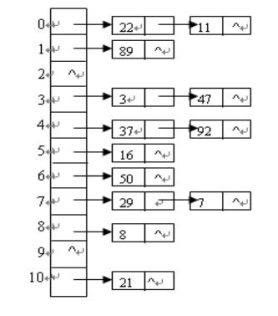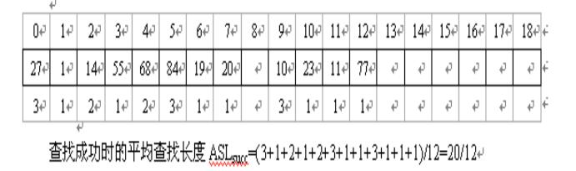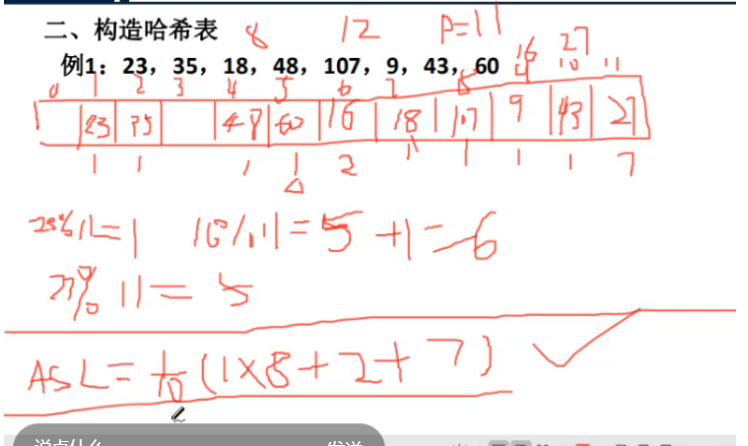# 你真的学会哈希表了么？

## 二.构造哈希表

1.直接定址法

H(key)=key 或H(key)=a*key+b


2 .数字分析法

3 .除留求余法

H(key)=key%p

p<=m
m 为哈希表的表长
p为不超过m的最大素数

## 三.处理冲突的方法

$H_i$=(Hash(key)+$d_i$) mod m i=1,2,…k (k<=m-1)

H(key)为哈希函数

m为哈希表长度

d i d_i 增量序列，可有下列3种取法：

• 线性探测再散列

d i d_i =1，2，3，…，m-1

• 二次探测再散列

d i d_i = 1²，-1²，2² ，-2²，3²，…，±k², k≤ m/2)

• 伪随机探测再散列

d i d_i ＝伪随机数序列

2.链地址法（拉链法 ）## 四.边学边练

H(19)=19%13=6

H(01)=01%13=1

H(23)=23%13=10

H(14)=14%13=1（冲突） H(14)=（1+1）%13=2

H(55)=55%13=3

H(20)=20%13=7

H(84)=84%13=6（冲突） H(84)=（6+1）%13=7（仍冲突）

H(84)=（6-1）%13=5

H(27)=27%13=1（冲突） H(27)=（1+1）%13=2（仍冲突）

H(27)=（1-1）%13=0

H(68)=68%13=3（冲突） H(63)=（3+1）%13=4

H(11)=11%13=11

H(10)=10%13=10（冲突） H(10)=（10+1）%13=11（仍冲突）

H(10)=（10-1）%13=9

H(77)=77%13=1205-2016万+07-0741
05-0637
04-1936
07-1720
10-2268
02-271989
08-15528
08-03137
01-0626
02-1096
09-01123
12-05
06-27
12-2777
10-15128
11-27241
06-29167
10-0814
11-29207